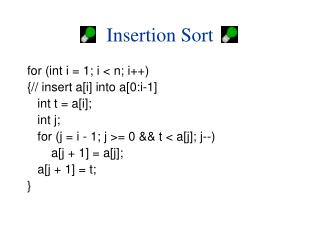DownloadDownload PresentationInsertion Sort

# Insertion Sort

Télécharger la présentation## Insertion Sort

- - - - - - - - - - - - - - - - - - - - - - - - - - - E N D - - - - - - - - - - - - - - - - - - - - - - - - - - -
##### Presentation Transcript

1. Insertion Sort for (int i = 1; i < n; i++) {// insert a[i] into a[0:i-1] int t = a[i]; int j; for (j = i - 1; j >= 0 && t < a[j]; j--) a[j + 1] = a[j]; a[j + 1] = t; }

2. Complexity • Space/Memory • Time • Count a particular operation • Count number of steps • Asymptotic complexity

3. Comparison Count for (int i = 1; i < n; i++) {// insert a[i] into a[0:i-1] int t = a[i]; int j; for (j = i - 1; j >= 0 && t < a[j]; j--) a[j + 1] = a[j]; a[j + 1] = t; }

4. Comparison Count • Pick an instance characteristic … n • For insertion sort, pick n = number of elements to be sorted. • Determine count as a function of this instance characteristic.

5. Comparison Count for (j = i - 1; j >= 0 && t < a[j]; j--) a[j + 1] = a[j]; How many comparisons are made?

6. Comparison Count for (j = i - 1; j >= 0 && t < a[j]; j--) a[j + 1] = a[j]; number of compares depends on a[]s and t as well as on i

7. Comparison Count • Worst-case count = maximum count • Best-case count = minimum count • Average count

8. Worst-Case Comparison Count for (j = i - 1; j >= 0 && t < a[j]; j--) a[j + 1] = a[j]; a = [1, 2, 3, 4] and t = 0 => 4 compares a = [1,2,3,…,i] and t = 0 => i compares

9. Worst-Case Comparison Count for (int i = 1; i < n; i++) for (j = i - 1; j >= 0 && t < a[j]; j--) a[j + 1] = a[j]; total compares = 1 + 2 + 3 + … + (n-1) = (n-1)n/2

10. Step Count A step is an amount of computing that does not depend on the instance characteristic n 10 adds, 100 subtracts, 1000 multiplies can all be counted as a single step n adds cannot be counted as 1 step

11. Step Count s/e for (int i = 1; i < n; i++)1 {// insert a[i] into a[0:i-1] 0 int t = a[i]; 1 int j; 0 for (j = i - 1; j >= 0 && t < a[j]; j--) 1 a[j + 1] = a[j]; 1 a[j + 1] = t; 1 } 0 for (int i = 1; i < n; i++) {// insert a[i] into a[0:i-1] int t = a[i]; int j; for (j = i - 1; j >= 0 && t < a[j]; j--) a[j + 1] = a[j]; a[j + 1] = t; }

12. Step Count s/e isn’t always 0 or 1 x = sum(a, n); where n is the instance characteristic has a s/e count of n (sum() is Program 1.30).

13. Step Count s/e steps for (int i = 1; i < n; i++)1 {// insert a[i] into a[0:i-1] 0 int t = a[i]; 1 int j; 0 for (j = i - 1; j >= 0 && t < a[j]; j--) 1 a[j + 1] = a[j]; 1 a[j + 1] = t; 1 } 0 for (int i = 1; i < n; i++) {// insert a[i] into a[0:i-1] int t = a[i]; int j; for (j = i - 1; j >= 0 && t < a[j]; j--) a[j + 1] = a[j]; a[j + 1] = t; } i+ 1 i

14. Step Count for (int i = 1; i < n; i++) { 2i + 3} step count for for (int i = 1; i < n; i++) is n step count for body of for loop is 2(1+2+3+…+n-1) + 3(n-1) = (n-1)n + 3(n-1) = (n-1)(n+3)

15. Asymptotic Complexity of Insertion Sort • O(n2) • What does this mean?

16. Complexity of Insertion Sort • Time or number of operations does not exceed c.n2 on any input of size n (n suitably large). • Actually, the worst-case time is Q(n2) and the best-case is Q(n) • So, the worst-case time is expected to quadruple each time n is doubled

17. Complexity of Insertion Sort • Is O(n2) too much time? • Is the algorithm practical?

18. Practical Complexities 109 instructions/second

19. Impractical Complexities 109 instructions/second

20. Faster Computer Vs Better Algorithm Algorithmic improvement more useful than hardware improvement. E.g. 2n to n3